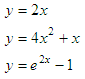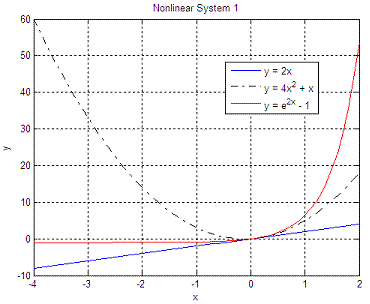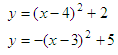# Nonlinear Simultaneous Equations

We’re going to develop a Matlab function to solve systems of nonlinear simultaneous equations.

 We’ll use the ‘fminsearch’ function to find the intersection of the given curves or functions with several variables. The method ends when a solution is found, when a maximum number of iterations is reached, or when some specifications of tolerances are met, according to the default options for fminsearch. For each system of nonlinear equations and starting

points, we need to be aware of the solution found, the number of iterations needed, the number of function evaluations needed, and the exit flag value. This helps us know whether the found solution is good or bad.

It’s important to note that this minimization or optimization function (fminsearch) doesn’t solve simultaneous equations. We have to adapt the system or formulate the problem in such a way that we can accomplish this. In this particular case, we’re going to express the nonlinear system as a scalar value, and it’s going to be reduced to its minimum value by fminsearch. That value will be the solution to our problem.

Naturally, different tolerances or starting points deliver different results. Only one intersection is found for every starting point, even though the nonlinear system may have more than one solution.

If we have this nonlinear system of simultaneous equations:We can express the system in Matlab as a function delivering a scalar:

function y = nls1(x)
y1 = 2 * x;
y2 = 4* x^2 + x;
y3 = exp(2 * x) - 1;

e1 = abs(y1 - y2);
e2 = abs(y2 - y3);
e3 = abs(y1 - y3);

y = max([e1 e2 e3]);

The important part is how we define our goal to be minimized. We want the differences of the functions to be as close to zero as possible. If the diferences of the functions (or errors between them) are not very close to zero, then we don’t have a good solution. We expect y to be zero at the end (where the curves intersect). 'fminsearch' will find the value of x that minimizes the maximum error between each pair of curves (that's called a minimax approach). Of course, we can formulate or express the system in different ways, this is only one idea. This function is called the ‘objective function’ (how we are going to measure the result), in numerical optimization terms.

Finally, we can minimize this type of systems with an optimization instruction. We pass two parameters: the name of the nonlinear system and the starting point (we’re using the default options, which can be modified with ‘optimset’).

fx = 'nls1';
x0 = -5
[x_sol, y_sol, EF, out] = fminsearch(fx, x0)

x_sol = 0
y_sol = 0
EF =    1
out =
iterations: 20
funcCount: 40
algorithm: 'Nelder-Mead simplex direct search'
message: [1x196 char]

This answer means that the intersection of the curves occurs at x_sol = 0, and y_sol = 0.

Now, we can try a different starting point, to compare solutions:

x0 = 10
[x_sol, y_sol, EF, out] = fminsearch(fx, x0)

The new answer is:

x_sol = 0
y_sol = 0
EF =    1
out =
iterations: 22
funcCount: 44
algorithm: 'Nelder-Mead simplex direct search'
message: [1x196 char]

Note that the solution in both cases is the same, but the number of function evaluations to get to the solution is not the same.

If the  curves  are very complex, with many ups and downs, the algorithm might not get a good answer. You should try then several starting points.

Another example. Let’s solve this system:Our ‘objective function’ is defined:

function y = nls3(x)
y1 = (x - 4)^2 + 2;
y2 = -(x - 3)^2 + 5;
y = norm(y1 - y2, 2);

The function is minimizing the absolute error between y1 and y2.

Each run finds only one answer. We must try different starting points to find different intersections. We run the script like this:

fx = 'nls3';
x0 = -20
[x_sol, f, EF, out] = fminsearch(fx, x0)

x0 = 15
[x_sol, f, EF, out] = fminsearch(fx, x0)

The two solutions are:

x_sol = 2.3820
f = 3.5793e-005
EF = 1
out =
iterations: 23
funcCount: 47

x_sol = 4.6180
f = 1.0069e-004
EF = 1
out =
iterations: 21
funcCount: 43

We find both solutions, x1 = 2.382 when we start at x0 = -20, and x2 = 4.618 when we start at x0 = 15.

From 'Nonlinear Simultaneous Equations' to home

From 'Nonlinear Simultaneous Equations' to Matlab Programming

 Top Linear Systems Intersections Curve Fitting